2

# Creating a Target Chart

by Richard Osbourn , VP Customer Success, Pyramid Analytics

# Background

It is helpful to analyze performance by creating a horizontal column chart that displays actual performance data against a target or goal.  The example below shows the following: product sales above target are displayed in green, product sales below target are displayed in orange, and the delta between actual and target (i.e., the shortfall) is displayed in grey.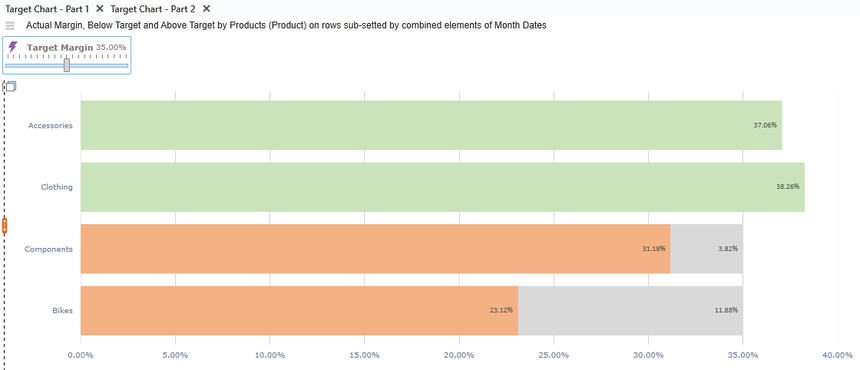A Target Chart Shows Performance Against Target

# Steps to Create

To demonstrate this example, the first step is to create a variable to represent the target value and allow the end user to change the target value to automatically see which products are under or over target.

The example is split into two parts: the first part is to calculate the Above and Below Target values, and the second part is to create the chart view.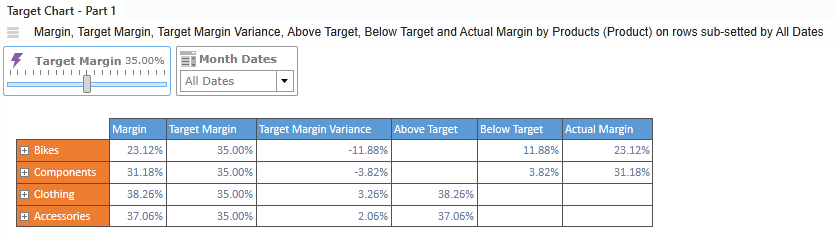Above and Below Target Values

## Part 1: Calculating Above and Below Target Values

For calculating the Above and Below Target values, the first step is to create a Custom Member in the Measures dimension equal to the variable value.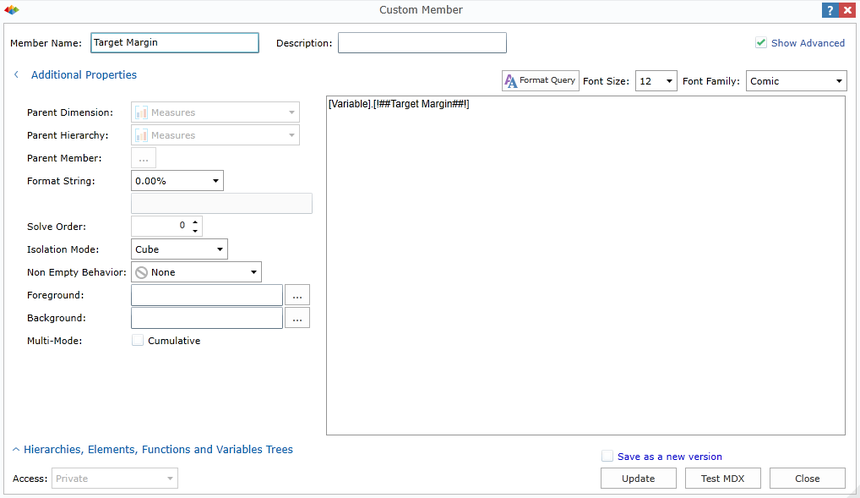Create a Custom Member Equal to the Variable Value

The next step is to calculate the Target Margin Variance which show the different between the Target and Actual value. In this step create a Custom Member in the Measures dimension; Target Margin Variance = Margin – Target Margin.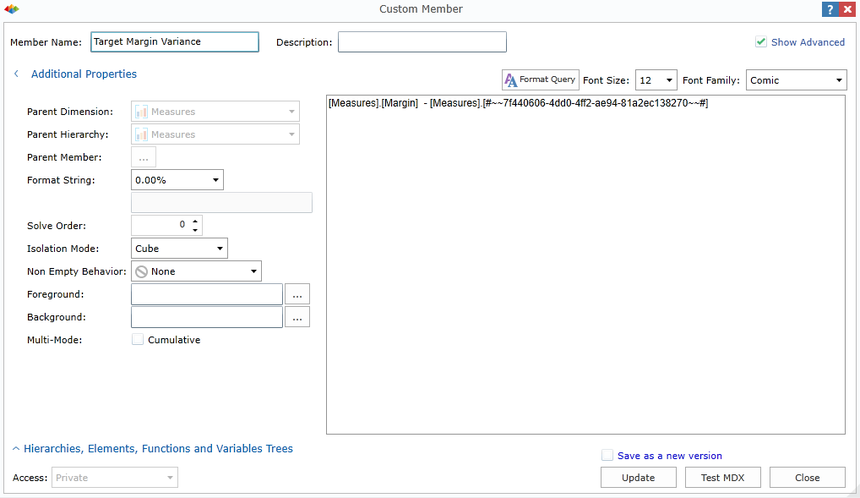Calculate the Variance

The next step is to create a Custom Measure in the Measures dimension called Below Target. The MDX statement uses an IIF statement to test which products are below target;

IF [Target Margin Variance] < 0 THEN [Target Margin Variance] * -1 ELSE NULL.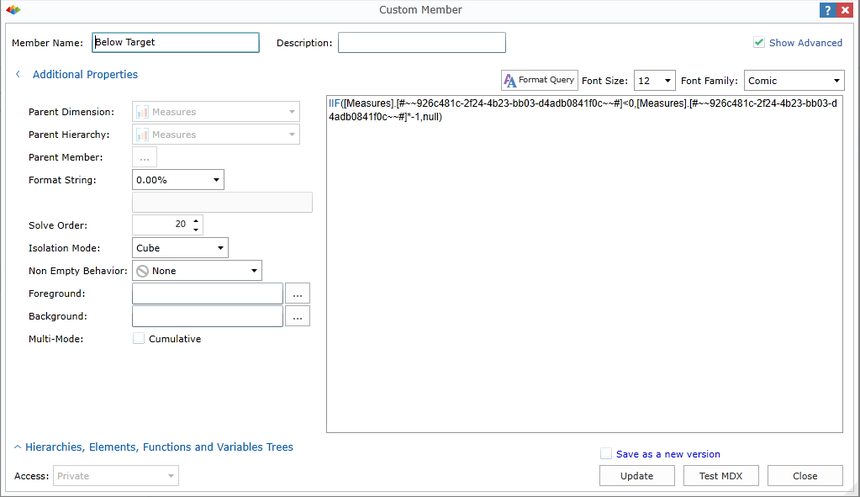Create the Below Target Custom Measure

Next, create a Custom Measure in the Measures dimension called Above Target. The MDX statement uses an IIF statement to test which products are above target;

IF [Target Margin Variance] > 0 THEN [Margin] ELSE NULL.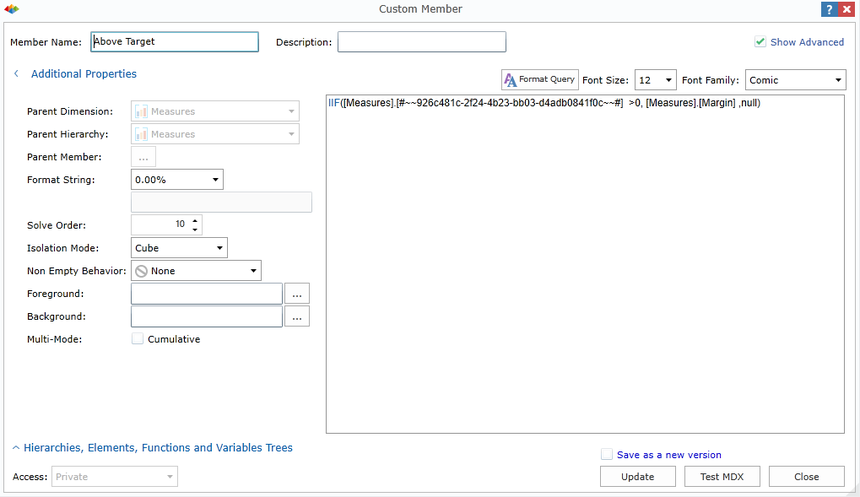Create the Above Target Custom Measure

The final custom measure created in the measures dimension is called Actual Margin.

If the margin is below Target then set the value equal to the Margin value, if not then set it to NULL.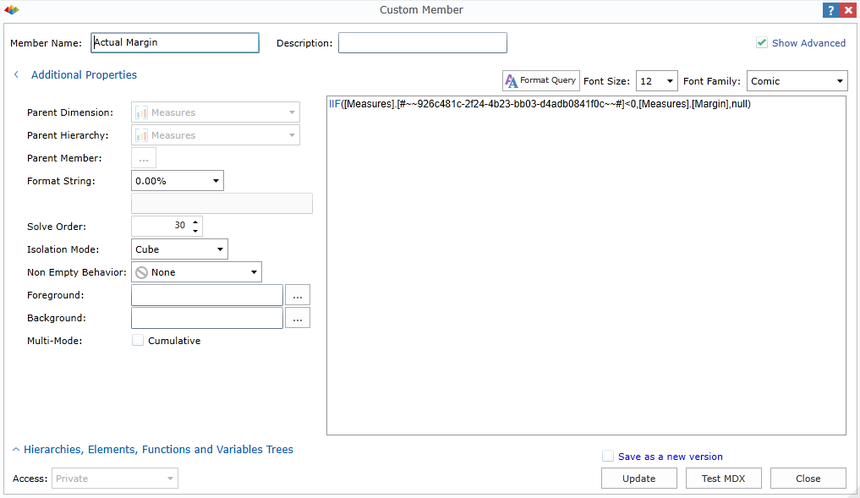Create the Actual Margin Custom Measure

## Part 2: Creating the Chart View

The second step is to create the chart view based on the customer measures: Actual Margin, Below Target, and Above Target.

The chart type should be set to a stacked horizontal column chart.

Edit each series to display the preferred column theme.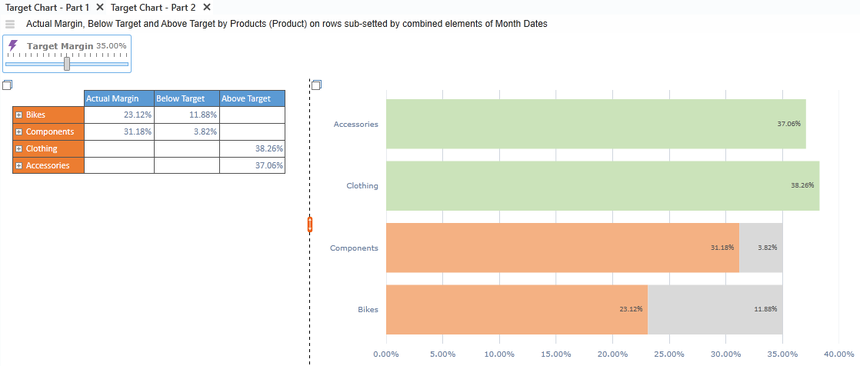A Stacked Horizontal Column Chart with Each Series Displaying a Column Theme